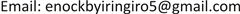Byiringiro Enock1,2

1武汉理工大学经济学院，湖北 武汉

2基加利独立大学经济学院，基加利1. 引言

2. 数据描述和ARDL模型2.1. 数据

Y t = α + γ PS t + Ω GCF t + Ψ FDI t + ε t (1)

2.2. ARDL模型

Pesaran等(2001)提出的自回归分布滞后(ARDL-Bounds)技术在检验整合变量I(0)或I(1)的长期关系时比其他方法更有效，对于小样本和大样本都能给出高效且可靠的测试结果。为了避免同阶整合问题和大量的观测需求，本文采用ARDL的模型如下：

Δ Y t = α 1 + ∑ i = 1 p 1 γ 1 i Δ Y t − i + ∑ j = 0 q 1 Ω 1 j Δ PS 1 t − j + ∑ k = 0 r 1 Ψ 1 k Δ FDI 2 t − k + ∑ g = 0 s 1 π 1 g Δ GCF 3 t − g + β 1 Y t − 1 + β 2 PS t − 1 + β 3 FDI t − 1 + β 4 GCF t − 1 + ε 1 t (2)

Δ Y t = α 2 ∑ i = 1 p 2 γ 2 i Δ Y t − i + ∑ j = 0 q 2 Ω 2 j Δ PS 1 t − j + ∑ k = 0 r 2 Ψ 2 k Δ FDI 2 t − k + ∑ g = 0 s 2 π 2 g Δ GCF 3 t − g + ω ECT t − 1 + ε 2 t (3)

Δ Y t = α 3 ∑ i = 1 p 3 γ 3 i Δ Y t − 1 + ∑ j = 0 q 3 Ω 3 j PS t − j + ∑ k = 0 r 3 Ψ 3 k FDI t − k + ∑ g = 0 s 3 π 3 g GCF t − 1 + ε 3 t (4)

3. 实证结果3.1. 时间序列的单位根检验

Unit root test result

Yt−4.586109***-−5.669523***-在I(0)水平平稳
PSt−2.717409*-−5.286530***在I(0)水平平稳
FDIt-−8.485796***-−8.935741***在一阶差分(1)平稳
GCFt−3.117398**-−3.117398**-在I(0)水平平稳

******分别表示拒绝原假设的单位根10%、5%和1%的显著性水平。

3.2. 边界检验结果

Boundary test result

F统计量12.039923

10%2.723.77
5%3.234.35
2.5%3.694.89
1%4.295.61

3.3. 协整性结果讨论

Long-term and short-term ARDL estimate

PS2.8410550.9437653.0103430.0395
FDI−1.7734260.379141−4.6774830.0095
GCF0.1993720.0275567.2351240.0019
C11.5729641.3359908.6624650.0010

CointEq (−1)−0.7981330.112700−7.0819110.0021
D (PS)−4.8039241.141215−4.2094820.0136
D (FDI)−1.4154290.253391−5.5859510.0050
D (GCF)0.2159630.01726012.5125980.0002

******分别表示拒绝原假设的单位根在10%、5%和1%的显著性水平。

3.4. 诊断性测试

4. 结论

Byiringiro Enock. 基于ARDL的卢旺达政治稳定与经济增长关系分析Analysis of the Relationship between Political Stability and Economic Growth in Rwanda Based on ARDL[J]. 世界经济探索, 2018, 07(04): 120-124. https://doi.org/10.12677/WER.2018.74014